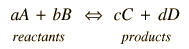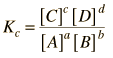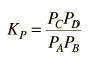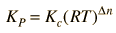# Chemical Equilibrium Constants

Chemical reactions may be envisioned in terms of reactants and products and written in the general formThe reaction may be spontaneous,in which case the reactants would continue to react until they are exhausted with the end composition being nearly all product. In other cases a significant amount of energy must be supplied to get the reaction to go at all, so that the reactants, left to themselves, would remain in their current form. In intermediate cases, there may be some particular mixture which will exist at equilibrium. It is in those cases where the idea of an equilibrium constant is of greatest value. The equilibrium constant may be expressed in the formwhere [C] represents the molar concentration of C at equilibrium. For a given reaction, the concentrations at equilibrium would have to be determined experimentally.

For gaseous reactants it is more convenient to express the equilibrium condition in terms of the partial pressures of the reactants and products. For this case the equilibrium constant is defined bywhere P denotes the partial pressure, usually in atmospheres. The two forms of the equilibrium constant are related bywhere Dn is the sum of the coefficients of the gaseous products in the chemical equation minus the sum of the coefficients of the gaseous reactants.

In application, there are practical cases where some of the reactants and/or products do not appear in the equilibrium constant expression. When there are pure solids or pure liquids involve, the concentrations are treated as one for these substances, so they do not affect the equilibrium constant.

The nature of the equilibrium constant allows us to make some qualitative judgments about the reaction. The general rules are:

1. If K>>1, the mixture will be mostly product.
2. If K<<1, the mixture will be mostly reactant
3. If K is about 1, the reaction will reach equilibrium at some intermediate mixture.
 Relation of K to electrode potentials
Index

Electrochemistry concepts

Reference
Ebbing
Ch 15

 HyperPhysics***** Electricity and Magnetism ***** Chemistry R Nave
Go Back